## Wednesday, March 19, 2014

### Inductive Reasoning Visualized

Ever wondered what inductive reasoning (technically, using inductive logic programming) would look like if you could draw a picture?
Here's how:

I'll explain what you're looking at. Let us say you know the following facts:
Gaia is a parent of Cronus.
Cronus is a parent of Zeus.
Zeus is a parent of Athena.

You also know that:
Gaia is a grandparent of Zeus.
Zeus is a grandparent of Athena.
Gaia is not a grandparent of herself.
Gaia is not a grandparent of Cronus.
Cronus is not a grandparent of Gaia.
Athena is not a grandparent of Cronus.

Now you ask the computer to induce a definition of grandparenthood based on these facts.

To do this, the machine needs to try different possibilities, and these are what you see in the graph.

On top, you see:
grandparent(_,_)
Now the sentence "X is a grandparent of Y" is what the machine writes as "grandparent(X,Y)", and an underscore simply means "anybody". So this is the hypothesis that "anybody is a grandparent of anybody".
The machine knows this to be false because of what you see in the red square: 4. Four is the number of "problems" found with this hypothesis: namely, it predicts that "Gaia is a grandparent of herself", which we know to be false. It predicts every instance of "is not a grandparent of" above, and there are 4 of them. Thus this hypothesis is not consistent with observations (its predictions are falsified).

Next we have
grandparent(A,_) :- parent(A,_)
which in English reads "A is a grandparent of anyone if A is a parent of anyone". As you can see, the red box says 3, because it has 3 problems: it predicts that Gaia is a grandparent of herself, since Gaia is a parent (of whom does not matter, but it happens to be Zeus), which we know to be false. For the same reason, it predicts that Gaia is a grandparent of Zeus, which is also false. Finally, it predicts that Cronus is a grandparent of Gaia, since Cronus is a parent (again, of whom does not matter). The negative example "Athena is not a grandparent of Cronus" is not (incorrectly) predicted to be true, since Athena is not a parent.

This is the basic idea in Inductive Logic Programming: we construct lots of hypotheses and test them against the examples we have. There are two solutions that look promising (green boxes):
grandparent(A,B) :- parent(A,C), parent(C,B)
which states that A is a grandparent of B if A is a parent of some C and that C is a parent of B. This is indeed the correct definition, and it does cover both positive examples ("Gaia is a grandparent of Zeus", "Zeus is a grandparent of Athena"), and does not cover any of the 4 negative examples.

The other promising solution is
grandparent(A,B) :- parent(A,C), parent(C,B), parent(B,_)
which gets the grandparent relation slightly wrong: on top of requiring the correct conditions (A is a parent of some C, which is a parent of B), it also requires the grandchild B to be a parent. So according to this definition, Athena is not the grandchild of Gaia because she does not (yet) have any children, but when she does, she'll satisfy the conditions. The machine knows this definition is worse than the right one because it cannot explain the fact that Zeus is a grandparent of Athena. Hence it only explains one of the two facts (that's the 1 in the green gox).

I'll leave it as an exercise to interpret all the other hypotheses in the graph.

The picture was produced using my ILP system Atom.

## The Isoperimetric Inequality

Given a rope of length L, say 100 meters, what is the largest closed area you can make?
If we try a square, each side has to be L/4 (25 meters), and we'll end up with an area of (L/4)^2 = 625 square meters. If we however try a circle, the circumference is L=2πR, where R is the radius. Thus R = L/(2π), and the area is π(L/2π)^2 = L^2/(4π) = 796 square meters, which is better than the square.

But there's plenty more shapes to try, in fact, infinitely many. So which should we pick to maximize the area?
The ancients knew the answer but could not prove it: it's the circle. The proof of this is surprisingly simple using calculus. Let L be the length of the rope, and let A be the enclosed area.

Note that for a circle, the relationship between A and L is L=2πR and A=πR^2, where R is the radius, so that: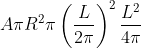Given the belief that the circle gives the largest area, we expect that for any shape,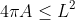This is known as the isoperimetric inequality.

## Hurwitz's Proof

Assume that the curve (x(t),y(t)) is parametrized so that it does one full revolution when t goes from 0 to π. The arc length is defined by s = Lt/(2π). Note that when t=0, s=0, and when t=2π, s=L.

We then have: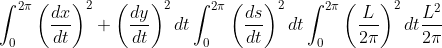As for the area, we use Green's theorem: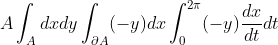Now, simply take the difference we want to show is always non-negative:We want to conclude that this expression is non-negative for any curve. The first integral is clearly non-negative, since its integrand is a square. As for the second integral, the result is not obvious until one consider its Fourier series (this is called Wirtinger's inequality):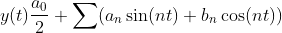As we will see soon, for this to work, we must place the curve in coordinates such that: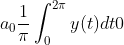This can always be done, as the placement of the curve is arbitrary: if the integral of y(t) is V, then the translated function y(t)-V/(2π) will have integral 0.

Next, differentiate y(t) to obtain: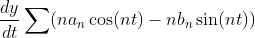Then, by Parseval's identity: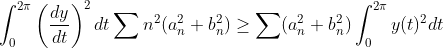which concludes the proof. (The requirement a0=0 is to ensure that the last inequality holds for n=0).
The inequality thus obtained is known as the isoperimetric inequality:## Only the Circle Yields Maximum Area

As we saw in the beginning, the circle turns the inequality into an equality, thus yielding the maximum possible area. But this still leaves open the possibility that other shapes may be equally good. We can show that only the circle yields max area by considering when the inequality becomes an equality: precisely when the two integrals are zero.

Starting with the second integral, the inequality was obtained from comparing the square sums after applying Parseval's identity. To have equality, the terms in those sums must be 0 except when n=1, which means that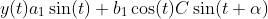The first integral must also be zero, which means that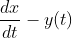Integrating, we get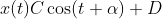So indeed we must have a circle centered at (D,0).

The fact that the circle must be centered around y=0 is due to our previous choice of fixing the integral of y(t) over one period. The center of mass must be such that equally much is positive and negative, which for a circle clearly means fixing it around y=0.

## How general is this proof?

The use of Green's theorem requires that we deal with a curve (x(t),y(t)) that has a continuous derivative except in finitely many points (piecewise smooth). Piecewise smoothness is also enough to ensure that the Fourier series can be differentiated term by term. Any area you can physically construct will certainly satisfy these conditions, as will anything you draw with a pen, since it is impossible to create or draw infinitely many edged points.

## Monday, October 21, 2013

### How to iterate over the values of a std::map

EDIT: Changed example to better reflect the generality of the problem = title of this post. END EDIT.

Now that C++ has lambdas, the C++ STL algorithms are practically useful, but how do you iterate over the values of a std::map?

Say you have a function template:

template <typename Iter> void f(Iter curr, Iter end);

f assumes the iterators point to std::strings:

std::vector<std::string> v;

f(v.begin(),v.end()); // ok


std::map<int,std::string> m;
f(m.begin(),m.end()); // Won't work: *m.begin() is a pair<Key,Value>

What we thus need is a way of converting the map::iterator (and map::const_iterator) to an iterator to always dereferences the Value type of the pair.

## Solution using Boost

The solution is easy using boost:

#define BOOST_RESULT_OF_USE_DECLTYPE
#include <boost/iterator/transform_iterator.hpp>

// First, define a lambda to get the second element of a pair:
auto get_second = [](const std::pair<const int,std::string>& p){ return p.second; };

// Then, we can convert a map iterator into an iterator that automatically dereferences the second element
auto beg = boost::make_transform_iterator(m.begin(),get_second);
auto end = boost::make_transform_iterator(m.end(),get_second);

f(beg,end); // ok, works!


The line
#define BOOST_RESULT_OF_USE_DECLTYPE
is needed so inform the boost libraries that the result type (Value in this case) should be inferred using decltype(), rather than by requiring a result_of typedef (prior to C++11, decltype did not exist).

## Solution without Boost

If you can't use boost, you'll need to implement the dereferencing by hand. Here's the code:

#include <map>
#include <iterator>

template <typename Iter>
class map_iterator : public std::iterator<std::bidirectional_iterator_tag,typename Iter::value_type::second_type> {
public:
map_iterator() {}
map_iterator(Iter j) : i(j) {}
map_iterator& operator++() { ++i; return *this; }
map_iterator operator++(int) { auto tmp = *this; ++(*this); return tmp; }
map_iterator& operator--() { --i; return *this; }
map_iterator operator--(int) { auto tmp = *this; --(*this); return tmp; }
bool operator==(map_iterator j) const { return i == j.i; }
bool operator!=(map_iterator j) const { return !(*this == j); }
reference operator*() { return i->second; }
pointer operator->() { return &i->second; }
protected:
Iter i;
};

template <typename Iter>
inline map_iterator<Iter> make_map_iterator(Iter j) { return map_iterator<Iter>(j); }

// We can now do:

 std::map<int,std::string> m;
 // ...
f(make_map_iterator(m.begin()),make_map_iterator(m.end()));






## STL Container Performance Table

There's already a good table at Stack Overflow listing time complexity (in Big O notation) of common operations with C++ STL containers for comparison, although it's structured in a more abstract way and a little hard to read because it's not a HTML table. Here's my version, which also includes priority_queue (technically an adaptor).

Persistent iterators means that the iterators are not invalidated by insertions and erase (except when erasing the element referred to by the iterator, which is necessarily invalidated).

ContainerInsertionAccessEraseFindPersistent Iterators
vector / stringBack: O(1) or O(n)
Other: O(n)
O(1)Back: O(1)
Other: O(n)
Sorted: O(log n)
Other: O(n)
No
dequeBack/Front: O(1)
Other: O(n)
O(1)Back/Front: O(1)
Other: O(n)
Sorted: O(log n)
Other: O(n)
Pointers only
list / forward_listBack/Front: O(1)
With iterator: O(1)
Index: O(n)
Back/Front: O(1)
With iterator: O(1)
Index: O(n)
Back/Front: O(1)
With iterator: O(1)
Index: O(n)
O(n)Yes
set / mapO(log n)-O(log n)O(log n)Yes
unordered_set / unordered_mapO(1) or O(n)O(1) or O(n)O(1) or O(n)O(1) or O(n)Pointers only
priority_queueO(log n)O(1)O(log n)--

## Always O(1): begin(), end(), empty(), size(), push_back()

The following operations are always O(1) when they exist:
1. begin(), end()
2. empty()
3. size() (note that list::size() was not necessarily O(1) prior to C++11)
4. push_front() (note that std::vector does not have push_front(), as it would not be O(1))
5. push_back()

For std::queue and std::stack, complexity depends on the underlying container used (by default std::deque).

### vector

std::vector has constant time (O(1)) back insertion provided no reallocation needs to take place (use reserve/capacity to allocate/check). When reallocation is necessary, all elements are copied (or moved, if possible) to a need memory location. It is guaranteed that back insertion is amortized constant, meaning: "if we perform a large amount of back insertions, the average time for back insertion is constant".

Insertion does not invalidate iterators as long as no reallocation is performed (when reallocating, all iterators become invalid). Deletion invalidates all iterators after the deleted element, iterators to elements before are still valid.

### deque

Insertion and deletion of elements in a std::deque may invalidate all its iterators. Pointers are however persistent. In practice accessing / iterating over a std::vector is faster than std::deque.

All iterators may become invalid after an insertion or deletion, but pointers/references are always valid.

### list

If you have an iterator to an element, you can insert right after (or before) that element in constant time (O(1)). Of course, you can also erase it or access it directly (O(1)) using the iterator (or any adjacent element, as ++iterator / --iterator are constant time operations).

If you only know the index, e.g. that you wish to insert/retrieve/erase the 4th element, you'll need to iterate the list until you reach that element. Put differently: std::list does not provide random access.

### sorted vector and deque

To search for an element in a sorted std::vector or std::deque, use std::equal_range. If only the first element is needed, there is std::lower_bound. If you only want to know whether an element exists or not, there is std::binary_search.

### set and map

Requires a less-than comparison function. Complexities also apply to multiset and multimap.

### unordered_set and unordered_map (hash tables)

unordered_set and unordered_map has constant time performance on all operations provided no collisions occur. When collisions occur, traversal of a linked list containing all elements of the same bucket (those that hash to the same value) is necessary, and in the worst case, there is only one bucket; hence O(n).

Requires a hash function and equality comparison function. Complexities also apply to unordered_multiset and unordered_multimap.

Deletion does not invalidate any iterators (other than erased element). Insertion keeps all iterators valid as long as no rehashing is done. When rehashing is performed, all iterators become invalid. Pointers/references to elements always remain valid.

### multiset, multimap, unordered_multiset, unordered_multimap

std::multiset and std::multimap follow the same rules as std::set and std::map.
std::unordered_multiset and std::unordered_multimap follow the same rules as std::unordered_set and std::unordered_map.

The only reason they are not listed in the table/throughout this document is to save space.

### basic_string

Strictly speaking std::string and std::wstring are typedefs for basic_string, which is a container. What applies to string above applies more generally to basic_string (and hence, to std::wstring too).

Note that prior to C++11 basic_string was not required to store its elements (characters) contiguously. Now it acts as std::vector, except its only valid for POD types and some tricks that don't violate the constraints may be employed (in practice: small string optimization).

### priority_queue

Requires a less-than comparison function. Always gives the greatest element (according to comparison function) when top() is called. top() is constant time, but pop() requires O(log n) as the queue needs to be rearranged (to ensure next top() correctly gives greatest element).

## Sunday, October 6, 2013

### Cracking Code Locks

When I was still a student in Linköping University, my friends were staying in student apartments where the main entrance used a code lock. The lock required a four digit passcode, but there was something unusual about it: it lacked the green "OK"/"Enter" button to confirm once choice. Instead, the code was automatically verified as the digits were inserted. This may not seem like such a big deal, but, as we will see, it is actually.

Consider that the code is 1234. Let us now say that you are returning home on a Saturday morning at 3.30 AM and wish to enter your building. Since you are not at your best, you happen to start by pressing a "2" instead of the "1". It's not such a big deal, you think, and you now press "1234", upon which the door opens. It works as you expected, but there is one crucial detail here: you actually inserted the string "21234", so the first four digits are in fact "2123", which is not the right code. When you then inserted the "4", the verification mechanism clearly knew that it should check the last three digits "123", and append your newly inserted "4".

Clearly then, you have just tried two code: "2123" and "1234", and you have in fact done so with only 5 key presses instead of the 4*2=8 you would normally expect. This is due to the missing "OK" button (and perhaps a "Cancel"/"Restart" button).

This convenience thus comes at a price: if someone wishes to crack the lock (by guessing the code that is, not physically breaking it), that cracker could reuse previously inserted digits as part of the new code. For instance, consider the following. First, we insert "0000", which tries precisely that code. Then, we insert a "1", upon which we are trying the code "0001". If we insert a "0" again now, we are trying "0010" (as the code lock is constantly remembering the last three digits inserted). We can clearly take shortcuts, but how many? If we could constantly insert new codes, without ever having to return to a previous code, we would effectively try all 10^4 = 10000 codes by pressing only 10003 digits (the 10000 codes + 3 digits we need to start the process).

At first glance, it's not even clear if it's possible to find such a sequence. Consider a code that does not deal with digits from 0 to 9, but only the binary 0 and 1. If the code is two digits long, then there are four different possible codes: 00, 01, 10, and 11 (not a very useful code lock for practical purposes, but it serves as an easy example for us to understand the problem). Normally, trying each code means we need to press 2*4=8 buttons (excluding the "OK" in between each). But if we don't have the "OK" button, so that the code lock uses a memory, we can in fact try all four codes by pressing "00110" (five presses instead of eight). To see why, consider each two consecutive digits in the sequence: 00, 01, 11, 10.

Now consider using binary digits again (only two buttons are available: 0 and 1), but with a code of length 3. We now have 8 possible codes: 000, 001, 010, 011, 100, 101, 110, 111. If we start with 000, we need to then append a 1 (otherwise we retry 000), so we begin with 0001. We could then start with 0 again, as the sequence 00010 would try 000, 001, 010 (just look at three consecutive digits in the sequence). Let's add another 0 and see what happens. The sequence is now 000100, which tries 000, 001, 010, 100. These are unique codes, which is great. However, we now run into problems, as the last 2 digits are 00, exactly what we began with. We have already tried both 000 and 001, so we are now forced into retrying a code!

The point is that some sequences will repeat previously tried codes, which is a waste of time. The sequences that do not repeat previous codes are known as De Bruijn sequences. So with binary digits and a length of 2, the sequence 00110 is a De Bruijn sequence, because it tries the codes: 00, 01, 11, 10, that is, all possible combinations exactly once each. The sequence 00011 is not a De Bruijn sequence, as it tries 00 two times (in the first two trials) and does not try the code 10.

Put differently, De Bruijn sequences are the shortest possible sequence of button presses needed to try all codes on doors with code memory (without "OK" button).

Do De Bruijn sequences exist for all number of digits and lengths (k and n)? We succeeded in finding such for binary digits with length 2 (k=2, n=2), but not for binary digits with length 3 (k=2,n=3). And what about the real world door codes, with has 10 digits and typically length 4 (k=10, n=4)?

It turns out that De Bruijn sequences exist for all possible digits k and length n. This means that a real world door code can be cracked in 10003 key presses instead of the expected 40000 (4 per code, 10000 codes). That is, it can be cracked four times faster!

To see that De Bruijn sequences always exist (and how to find them), we first make some observations:
1. At any point, we have a state, which are the n-1 digits previously inserted (the "memory").
2. We then make k different choices, each giving us a code. Put differently: at each point (after initializing with n-1 digits), we have n-1 digits in state and we choose one more digit (k choices) to obtain a new code of length n.
3. To get a code, say 1234, there is in fact only one way to reach it: from the state 123, and adding the digit 4.
We can picture this process in a graph, where the nodes represent states, and the links represent codes. Thus, note that attempts to try a code are on the links (edges), not nodes (vertices). Below you can see such a graph for k=2 (only binary digits) and n=4 (all codes are composed for four binary digits). The correct code might thus be e.g. 1001 or 0111.

Clearly, the question is now: can we traverse this graph in a way that passes each link once and only once? Note that we may be in the same state multiple times. In fact this is always necessary: to try both 0000 and 0001, we need to be in the state 000 first. However, we don't want to try any code more than once, so each link should only be visited once. This is known as a Eulerian path (or cycle, if we finish at the same node as we started). Now, each node in the graph will have exactly k links going out and k links going in (k=2 in the depicted graph), since there are k digits to choose from in each state, and k ways to throw away a digit to obtain the state. For example, in the graph above, there are two ways out of the state 000: by adding another 0, or by adding a 1. This corresponds to trying code 0000 and 0001. There are also two ways to reach 000: from 0000, and from 1000, as we throw away the left most digit (oldest digit).

Since there are equally many links pointing in and out (namely, k), we can be sure there is an Eulerian cycle, so our problem is indeed always solvable! In fact, all solutions are given precisely by all Eulerian cycles, so any algorithm to find cycles will do (Hierholzer's algorithm is not only an efficient and intuitive way of finding such cycles, but also provides a proof of existence).

### Cracking Suitcases

Cracking suitcases is similar (k=10, n=3), except that we can now rotate each wheel independently. Thus, in one "click" we can go from 000 to 001, 010, 100, 009, 090, and 900. These six "next codes" corresponding to rotate one of the three wheels one step "up" or "down" (to their adjacent values). If we were to depict the solutions as a graph, where each node again corresponds to state and links to solutions, we have an important difference: the state is now the previous solution (we don't throw away any digits). From each state there are 6 links going out, and in fact, all these links are also going in, since we can always turn the wheel back one step. Thus we may consider the undirected graph where each node has 6 links, and, since every node has an even degree, it is guaranteed that an Eulerian cycle exists.

Note that the "obvious" solution of enumerating does not work: 000, 001, 002, 003, ... 008, 009 is fine, but going from 009 to 010 requires two switches: turning the least significant 9 to 0, and the middle 0 to 1.

In this manner, we only need to rotate the "wheels" a total of 1000 times, as each rotation tries a new code (and there are precisely 1000 codes, namely 000 to 999).

## Thursday, September 26, 2013

### Measuring Execution Time Accurately and Setting Deadlines

If you do benchmarking, a common task is to measure the execution time of a particular block of code.

The canonical and portable way to do this in C++ is as follows:

#include <chrono>
// Starting timepoint
// ... Code here ...
double time_in_seconds = std::chrono::duration_cast<std::chrono::milliseconds>
(std::chrono::steady_clock::now() - start_time).count() / 1000.0;

This will measure execution time in seconds, with millisecond precision. If you want better precision, you can of course go for say microsecond resolution:

// Starting timepoint
// ... Code here ...
double time_in_seconds = std::chrono::duration_cast<std::chrono::microseconds>
(std::chrono::steady_clock::now() - start_time).count() / 1000000.0;

If your code block may throw exceptions, and the execution time is actually updated to some variable that is outside of this scope, you may want to catch the exception:

// time_in_seconds reference to variable declared outside this scope
// Starting timepoint
try {
// ... Code here ...
} catch (...) {
// Update execution time and rethrow
time_in_seconds = std::chrono::duration_cast<std::chrono::milliseconds>
throw;
}
// Normal execution ended, update time
time_in_seconds = std::chrono::duration_cast<std::chrono::milliseconds>
(std::chrono::steady_clock::now() - start_time).count() / 1000.0;

steady_clock is a monotonic clock: its value never decreases. This can be compared to std::chrono::system_clock, which can in fact decrease if the user changes the value. There's also std::chrono::high_resolution_clock which may or may not be monotonic (as the name suggests, it is intended to primarily be used as a high resolution clock, i.e. ideally with nanosecond precision).

The start_time is a timepoint, and taking the difference of two timepoints (the two now()s) yields a duration. We then convert the duration into millisecond "ticks", which are obtained using count(). Alternatively, we can convert the duration into microsecond "ticks" (as shown above), or even nanosecond ticks. Finally, we refactor the numerical value from milliseconds to seconds (this step is obviously not needed, but is used because it is many times easier to use SI units).

Deadlines can be created in a similar way:

// Define type used for deadline

// or, equivalently: now() + std::chrono::seconds(2*60);

for (const auto& e : vec) {
if (std::chrono::steady_clock::now() > soon) throw out_of_time();
// ... process vector elements ...
}





## Monday, August 19, 2013

### Fast (and pretty easy) Currency Conversion without Calculators

With the prevalence of mobile phones, it's easy to convert between currencies by simply using the phone's calculator. If you're like most people, however, you won't be doing this while shopping on the street markets of Thailand or anywhere else abroad, provided the sum is not too large (say anything below 100 USD).

Here's a practical way to convert between currencies fast and easy, all done in your head.

Consider some practical examples.

USD to EUR conversion
We want to convert from USD to Euros (EUR).
Google tells me that today, 1 USD = 0.75 EUR.
How much is 67 USD in EUR?

Note that 0.75 = 3/4, so we have 67 * 3 / 4. From a mathematical point of view, it doesn't matter if we multiply 67 by 3 first, and then divide it by 4, or if we divide 67 by 4 first, then multiply by 3. It is however much easier from humans (and computers!) to deal with small numbers, so we start by dividing to get a smaller number.

How do you divide 67 by 4? The answer is that you half it two times: 67 / 4 = 67 / (2*2) = 67 / 2 / 2.
How do you halve 67? 67 is 60+7, and if we take half of each and then add them, we'll have the correct answer. Half of 60 is 30, and half of 7 is approximately 4 (we stick to integers). Thus the answer is 30+4 = 34. Repeating once more (remember, we halve two times), 34 = 30+4, and halving each gives 15+2 = 17.

Now, we multiply 17 by 3 using the same technique of splitting 17 into 10+7 and then multiplying (instead of dividing) by 3: 3*10 = 30, and 3*7 = 21, so you get 30+21 = 51.

In one go: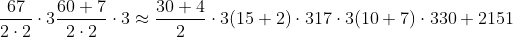67 USD is approximately 51 EUR. The exact answer is 50.25 EUR.

What you need to remember is thus:
1. The general operations: halve the number two times, then multiply by 3
2. How to halve and multiply by 3 using the "splitting" trick.
Let's we want to do the opposite now: convert euros into USD. 1/0.75 = 1.33, so 1 EUR = 1.33 USD.

Let's say we have 247 EUR. This is 247*1.33 USD, but how do we calculate it easily?

First, we omit the second decimal, i.e. 1.33 is approximately 1.3. So we only need to do 247 * 1.3. First, using the splitting trick, we see that: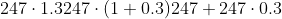So the hard part is a little easier now: we need to estimate 247*0.3. Noting that 0.3 = 3/10, and doing the division before multiplication as before, we get: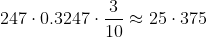So the answer is 247 + 75. A rough estimate is given by 250+75 = 250 + 50 + 25 = 325.

So 247 EUR is approximately 325 USD. The exact answer is 328.51 USD.

It seems then that the trick is to come up with ways to multiply and divide by decimal numbers, such as 0.75 and 0.3. This can always be done, since all conversion rates are rational numbers, meaning they can be written in the form Integer/Integer. By using a fairly simple numerator and denumerator, we can then perform the estimation fast. Multiplication is in general easy, division much harder. Here's a couple of tricks:

Divide by 2 (halving)
This is simply taking half the quantity. If you need to divide say 573 by 2, then use the splitting technique: 573 = 500 + 70 + 3. Halving each gives approximately 250 + 35 + 2 = 250 + 37 = 287.

Divide by 4 and 8 (halving many times)
Also very easy: dividing by 4 is the same as halving twice. Dividing by 8 is the same as halving three times.

Divide by 10
The easiest to do. 128 divided by 10 is 12.8. In general, XYZ divided by 10 will be XY.Z, where X,Y and Z are digits (0,1,2,3,...,9). We then simply round to nearest integer> 12.8 is closest to 13.

Divide by 5 ("divide by 10 and double")
This one's really easy too: x/5 = x/10*2, so all you need to do is divide by 10 (using the method above), and then double the result. Example: 128/5 can be calculated first dividing 128 by 10, giving 13. Doubling gives 26.

Divide by 3 ("take average of 2 and 5")
This one's a bit harder. A nice way to estimate x/3 that builds on the techniques already mentioned is to take the average of x/2 and x/5.
Example: 175 divided by 3 can be approximated by noting that:
175/5 = 18*2 = 36
175/2 = (100 + 75 + 5) / 2 = 50 + 37 + 3 = 50 + 40 = 90
Taking averages, we get (36+90)/2 = 126/2 = (100+20+6)/2 = 50 + 10 + 3 = 63.
In general, this technique gives (x/2 + x/5)/2 = 7x/20 = 0.35x, instead of 0.3333x.
(It may seem more intuitive to take average of x/2 and x/4, but this gives a worst estimate of 0.375x.)

Divide by 7 ("take average of 5 and 10")
Also a bit harder. When doing x/7, we can take the average of dividing by 5 and 10.
384/7 is somewhere between:
384/10 = 38
384/5 = 38*2 = (30+8)*2 = 60 + 16 = 76
Taking averages, we get (38+76)/2 = (100 + 14)/2 = 50 + 7 = 57
The exact answer is close to 55.
This techniques calculates (x/5 + x/10)/2 = 3x/20 = 0.15x instead of x/7 = 0.143, so it's a good estimate for relatively small sums of money.

Here's another example. 1 THB (Thai Baht) is 0.032 USD. How much is the saleswoman asking for in USD when she says 650 THB? The answer is 650*0.032 USD. To estimate this quantity, we manipulate the conversion rate 0.032 into rules of the form above. Note that 0.032 = 32/1000 which we approximate as 30/1000 = 3/100 (32/1000 is (30+2)/1000 = 3/100 + 2/1000, so we're skipping a term containing two parts in 1000).

Calculating 650 * 3/100 is easy: do the division first (dividing by 100 is dividing by 10 two times, since 100 = 10*10). We then get 6.5, which we round to 7. Multiplying by 3 gives 21. Here's the full estimate in one line: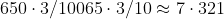Let's now convert from GBP (Brittish pound) to USD. 1 GBP = 1.56 USD. How much is Harrods asking you in USD when they say 489 GBP?

First we deal with the conversion rate. 1.56. This is close to 1.6, which we instead use. 1.6 = 16/10, and looking at prime factors we have: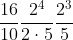This works: we can divide by 5 and then double 3 times. It's however rather tedious, as if we allow a little more rounding error, 1.56 is also close to 1.5, which gives: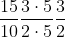A much simpler calculation: we half, then multiply by 3.
Thus, estimating 489 GBP is done as follows:
489/2 = (400 + 80 + 9)/2 = 200 + 40 + 5 = 245.
245 * 3 = (200 + 40 + 5)*3 = 600 + 120 + 15 = 720 + 15 = 735.

489 GBP is approximately 735 USD (although for such large amounts I would suggest you use a calculator!).